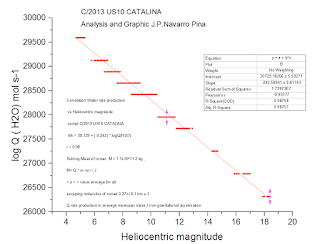## viernes, 29 de abril de 2016

### MASS , DIAMETER , WATER PRODUCTION , AND ANALYSIS OF THE LIGHT VISUAL CCD OF COMET C/2013 US10 CATALINA

Recent update of the light visual ccd curve based in 2430 ccd's-visual observations frome the database MPC/IAU , i calculated the new photometrical's parameters and i have discovered 3 different photometric laws that dominate the general curve photometric comet , the water rate production max. is 4000 kg/sec. log Q ( H2O ) = 10^29.6 mol sec , the mass estimated is log M (kg ) =11.2 , M = 1.1x10^11 kg , diameter calculated D(kms ) = 0.96 . graphic and analysis down . The general rate production of comet C/2013 US10 CATALINA , ( Jorda et al. 2008 ) [ log Q = -0.8 / n * m0 + C , Result log Q = -3.721 + C ]### Update Analysis Light Curve C/2021 A1 LEONARD A1

My second analysis of the light curve of comet C / 2021 A1 LEONARD, indicates in T-300, a stagnation in the rate of increase in brightness ...